Pivot tables
The totals function sums the values in columns or rows within a pivot table.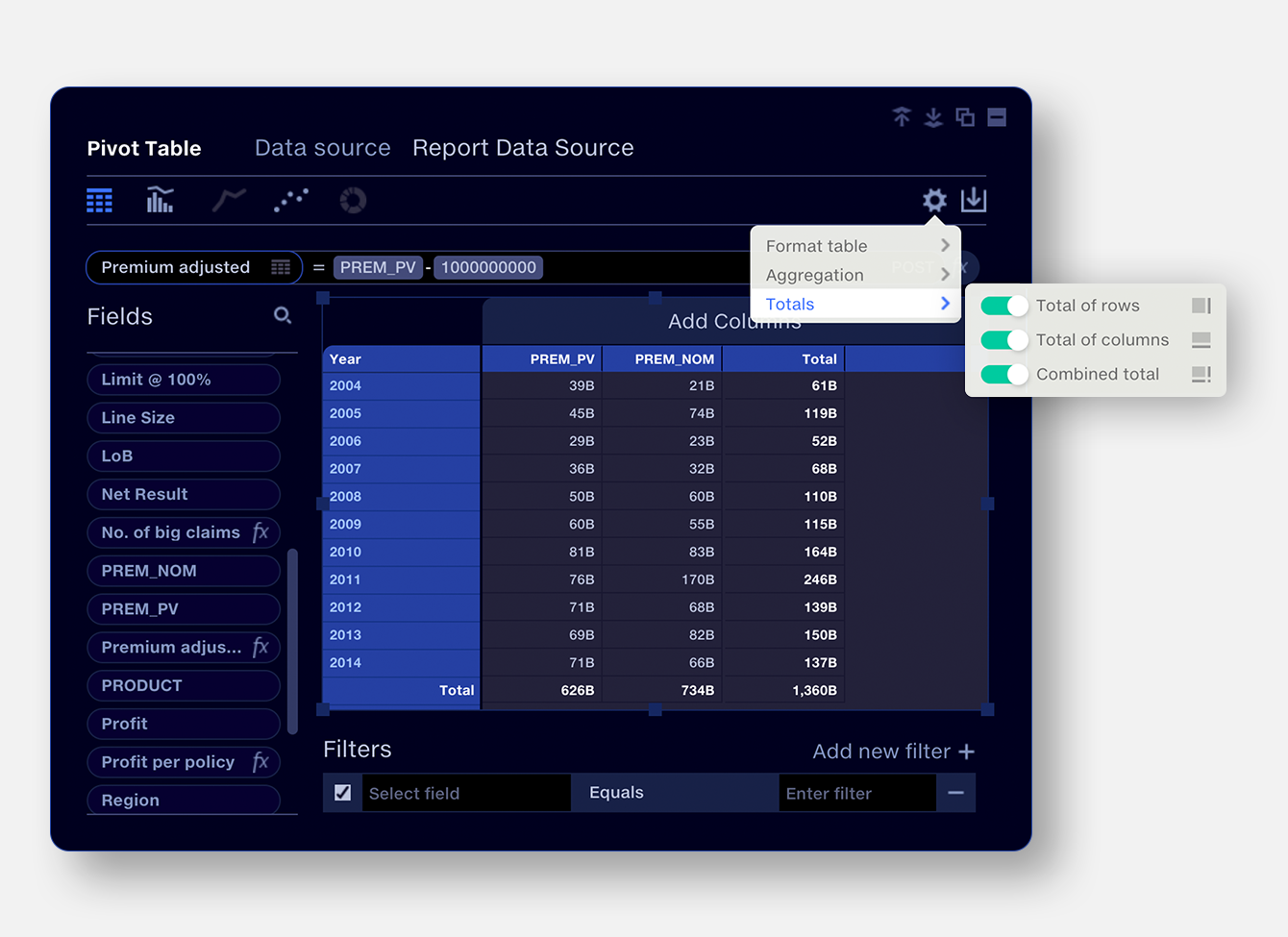Total of rows

The sum of the values for each row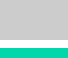Total of columns

The sum of the values for each column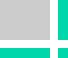Combined total

The sum of every value in the table. Row and column totals must be enabled to show combined totals.

Totals support sum and count aggregations in the pivot table. If other table aggregation settings are selected, totals will be disabled.
Repeated fields
Value fields which are included more than once in a pivot table are counted only once in the total. This is to allow different formatting of the the same value field (e.g. formatting as ‘% of total’) without affecting the total. Additionally, if a calculation contains only a value field, and that value field is already in the pivot table, it will not be included in the total.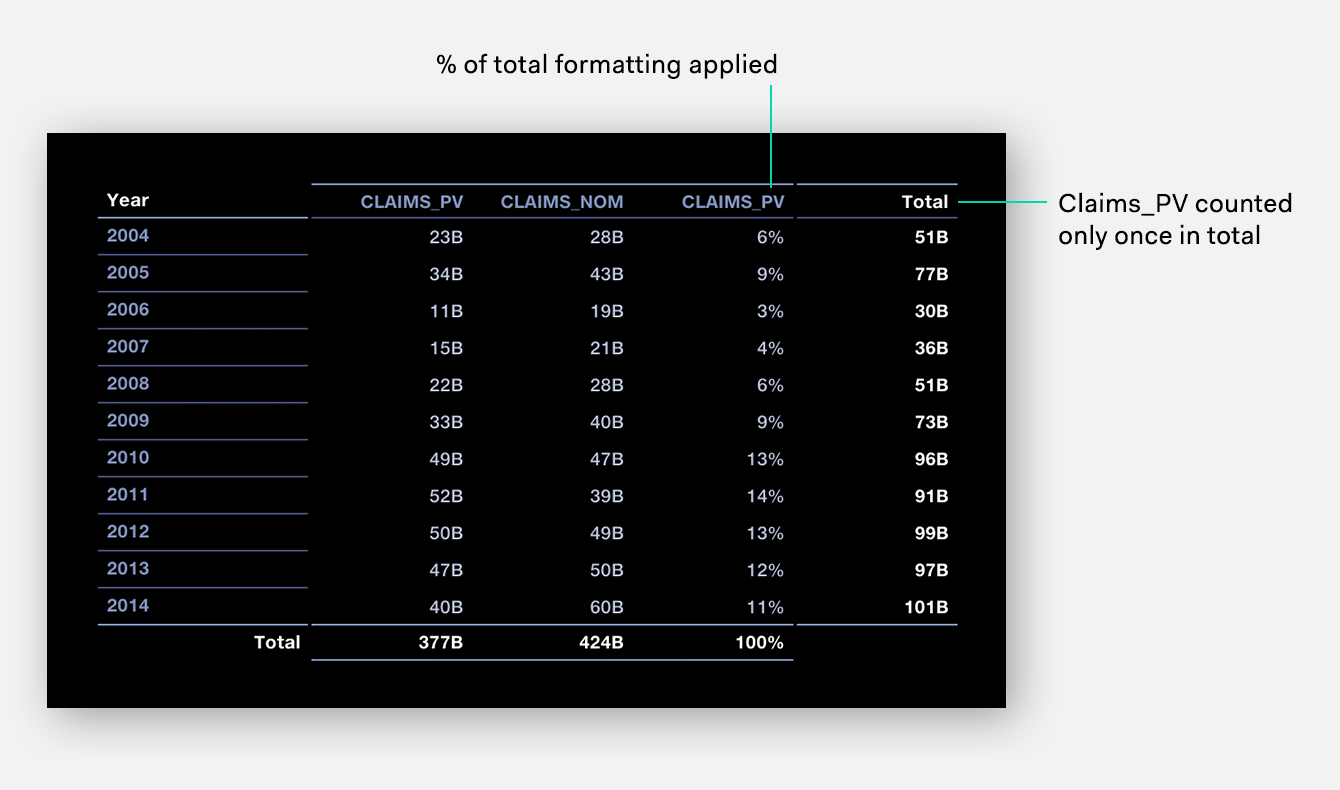Totals and calculated fields
Totals for pre- and post-pivot equations are calculated differently. This is so that non-additive calculations such as Loss Ratios, which are typically performed post-pivot, are calculated correctly.

For pre-pivot equations, the equation is applied to each row of the source data, before being aggregated in the pivot table. The total is the sum of these calculated values.
``````Pre-pivot equation: A/B
Total = ∑ (A/B)``````
For post-pivot equations, the equation is applied to the aggregated values in the pivot table. The total is calculated from the totals of the categories referenced in the equation.
``````Post pivot equation: A/B
Total = ∑A/∑B ``````
Example: pre-pivot equation
A calculated field ‘Premium adjusted’ is created subtracting 10,000 from each row in the ‘Premium’ column of the source data.

``39+45+28+… = 615``Example: post-pivot equation
A calculated field ‘Loss ratio’ is formulated from the fields ‘Claims’/‘Premium’ and set to post-pivot. The values appearing in each row of the ‘Loss ratio’ column are calculated from the aggregated Claims and Premium values for each year.

The total of ‘Loss ratio’ is calculated from ∑ ‘Claims’/ ∑ ‘Premium’, rather than summing the individual rows of the ‘Loss ratio’ column.
``````∑'Claims' = 377
∑'Loss ratio' = 377/626 = 60%``````Note that post-pivot equations containing predicates, such as IF statements logically cannot be totalled, so will just display a ‘–’ in the total row.
Totals and calculations containing constants
Pre-pivot calculations containing constant values (eg ‘10,000’) work as in the pre-pivot example above.

Post-pivot calculations where a constant value is added or subtracted apply the rules outlined in the Post-pivot example above. The total is calculated from the aggregate values.

This may give the appearance of an unexpected total result, so caution is advised when using totals for post-pivot calculations which add or subtract constant values.

Post pivot calculations using the operators / * ^ will appear normal.
Example: Post-pivot equation subtracting a constant value
You wish to subtract 1Bn from each annual aggregated premium.
``'Prem adjusted' = 'Premium - 1000000000' set to post-pivot.``
The total for ‘Prem adjusted’ will be:
``'Prem adjusted' = ∑ 'Premium' - 1000000000  ``
Note that this is a different result from the Sum of all values in ‘Prem adjusted’ column.Totals and number formatting
Totals adopt the same number formatting as the values. If values with multiple number formats are contained in a table, the total will use the same formatting as the first value field from the left or top.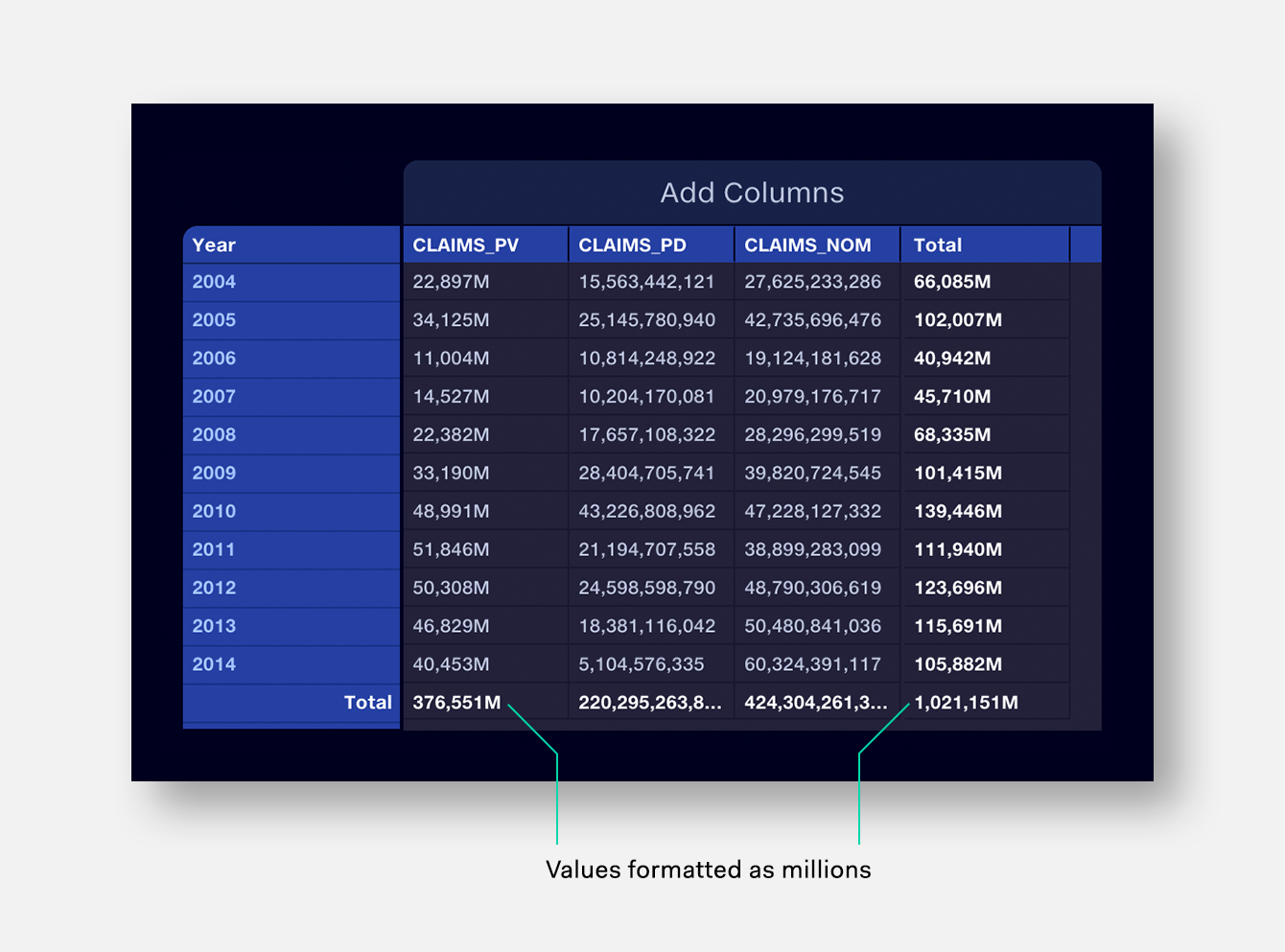If values are set to display to a certain magnitude or number of decimal places, totals are still calculated from the raw data, before the formatting is applied, avoiding rounding errors.